DeclaraCAD has `Fuse`, `Cut`, and `Common` boolean operations.

Note: Use `parallel = True` to run the operation in parallel. Use `disabled = True` to quickly disable the operation and make the result the first shape.

### Fuse

The `Fuse` declaration performs a boolean add or union of two shapes. The resulting shape will be both parts fused together as if welded, casted, or printed as one piece. `Fuse` will join all child declarations so multiple shapes can be fused together with one `Fuse` block.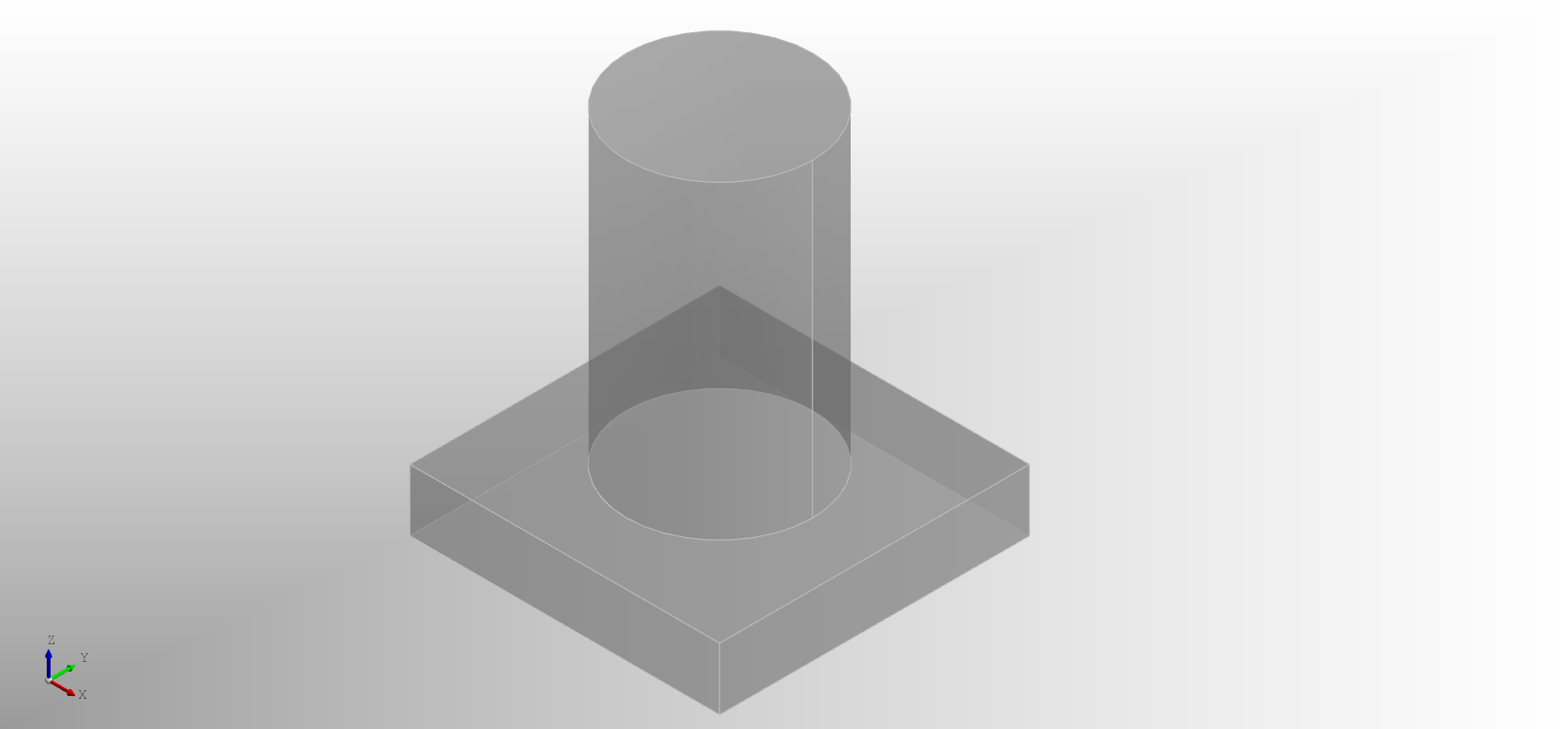``````enamldef Assembly(Part):
Fuse:
transparency = 0.5
material = 'plastic'
color = 'grey'
Box:
position = (-dx/2, -dy/2)
dx = 5
dy = 5
dz = 1
Cylinder:
position = (0, 0, 1)
height = 5
``````

Note: When performing a union of planar faces OCCT will keep each original face intact. You can set the `unify = True` to join all of the faces into a single face as shown below (on the left).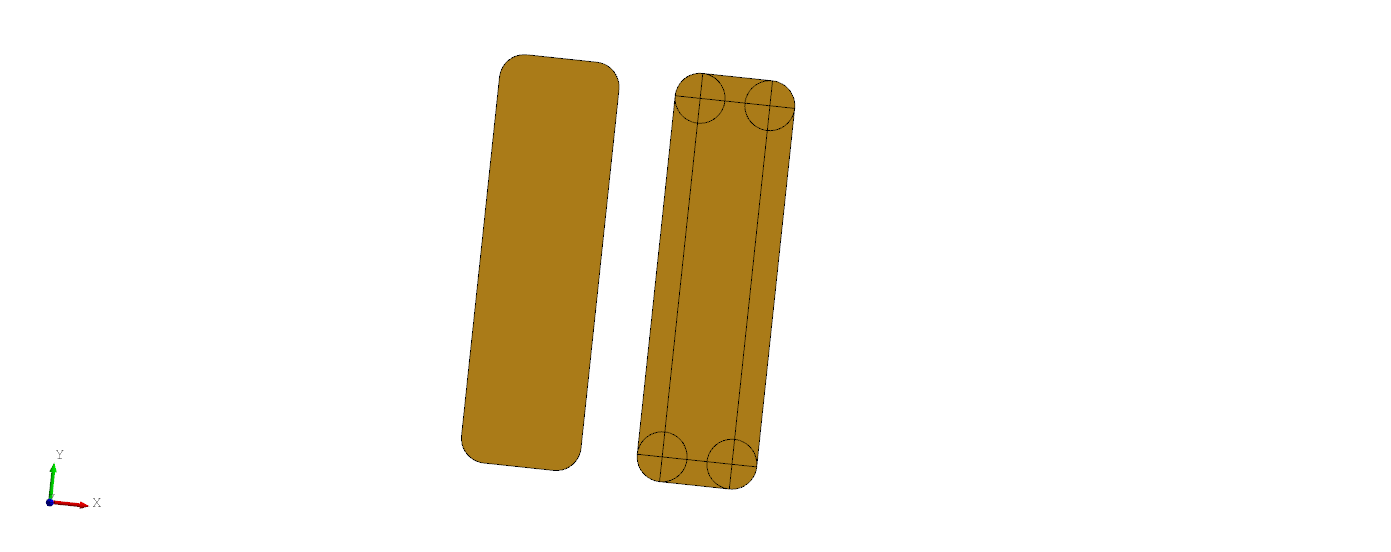### Cut

The `Cut` performs a subtraction of the all subsequent shapes from the first shape. The resulting shape will be the first shape with all other intersecting parts of the other shapes removed.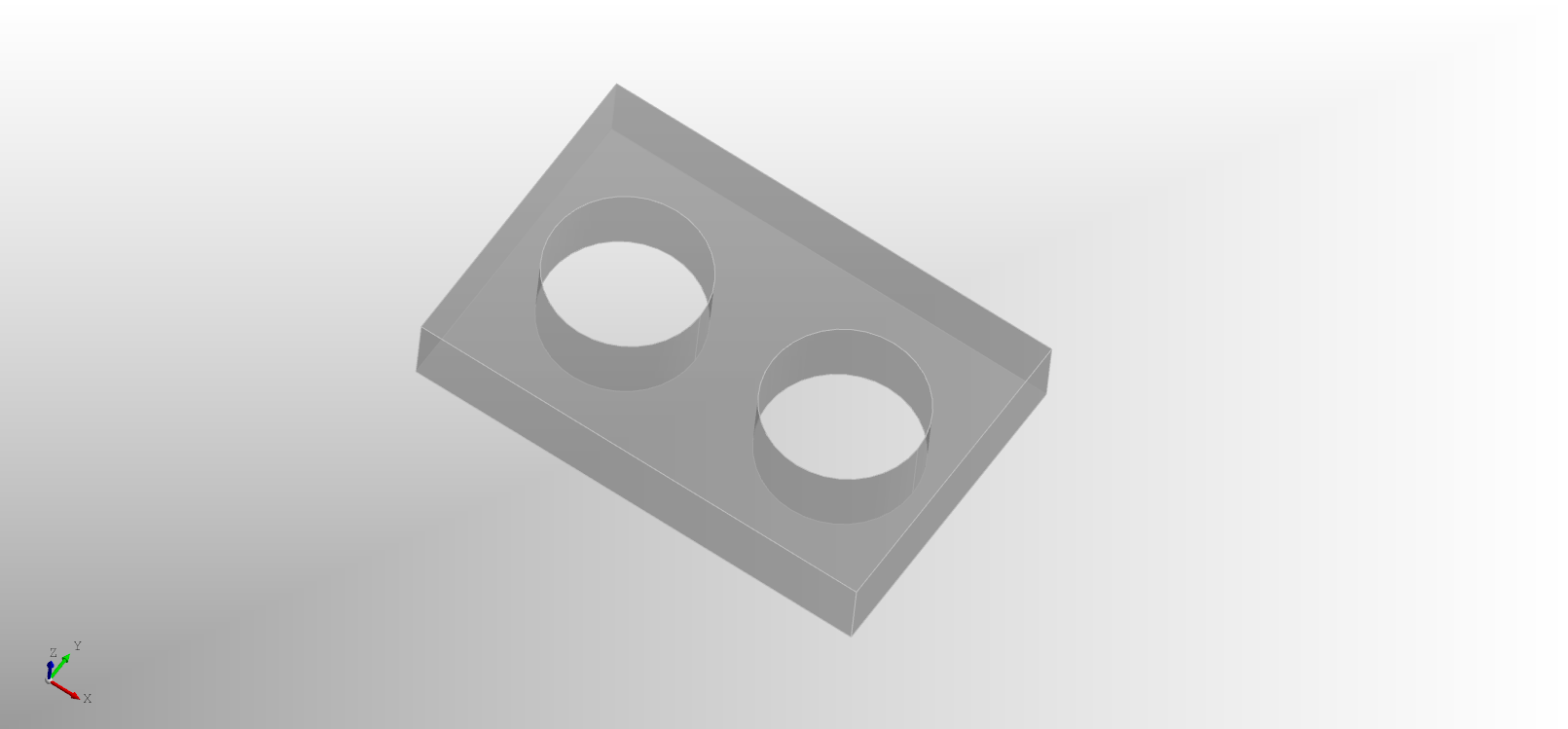``````enamldef Assembly(Part):
Cut:
transparency = 0.5
material = 'plastic'
color = 'grey'
Box: box:
position = (-dx/2, -dy/2)
dx = 6
dy = 4
dz = 1
Cylinder:
position = (1.5, 0)
height = box.dz
Cylinder:
position = (-1.5, 0)
height = box.dz
``````

### Common

The `Common` performs an intersection of all of the shapes. The resulting shape will be only parts which are common to all of the items.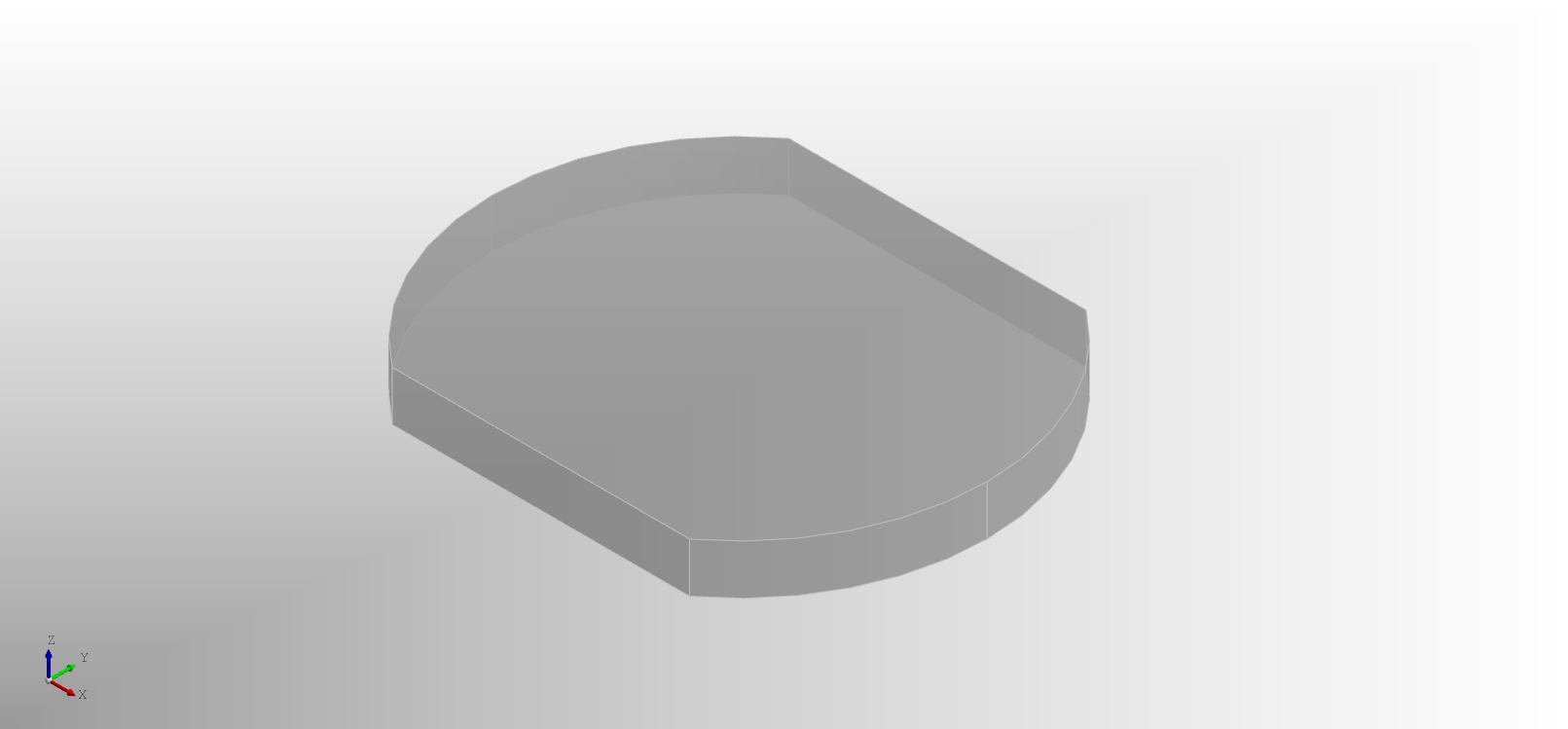``````enamldef Assembly(Part):
Common:
transparency = 0.5
material = 'plastic'
color = 'grey'
Cylinder:
position = (0, 0)
height = 1
Box:
position = (-dx/2, -dy/2)
dx = 10
dy = 8
dz = 1
``````

Any combination of these operations can be nested together as needed to create more complex parts. As an example here's one way to model a simplified ball screw nut.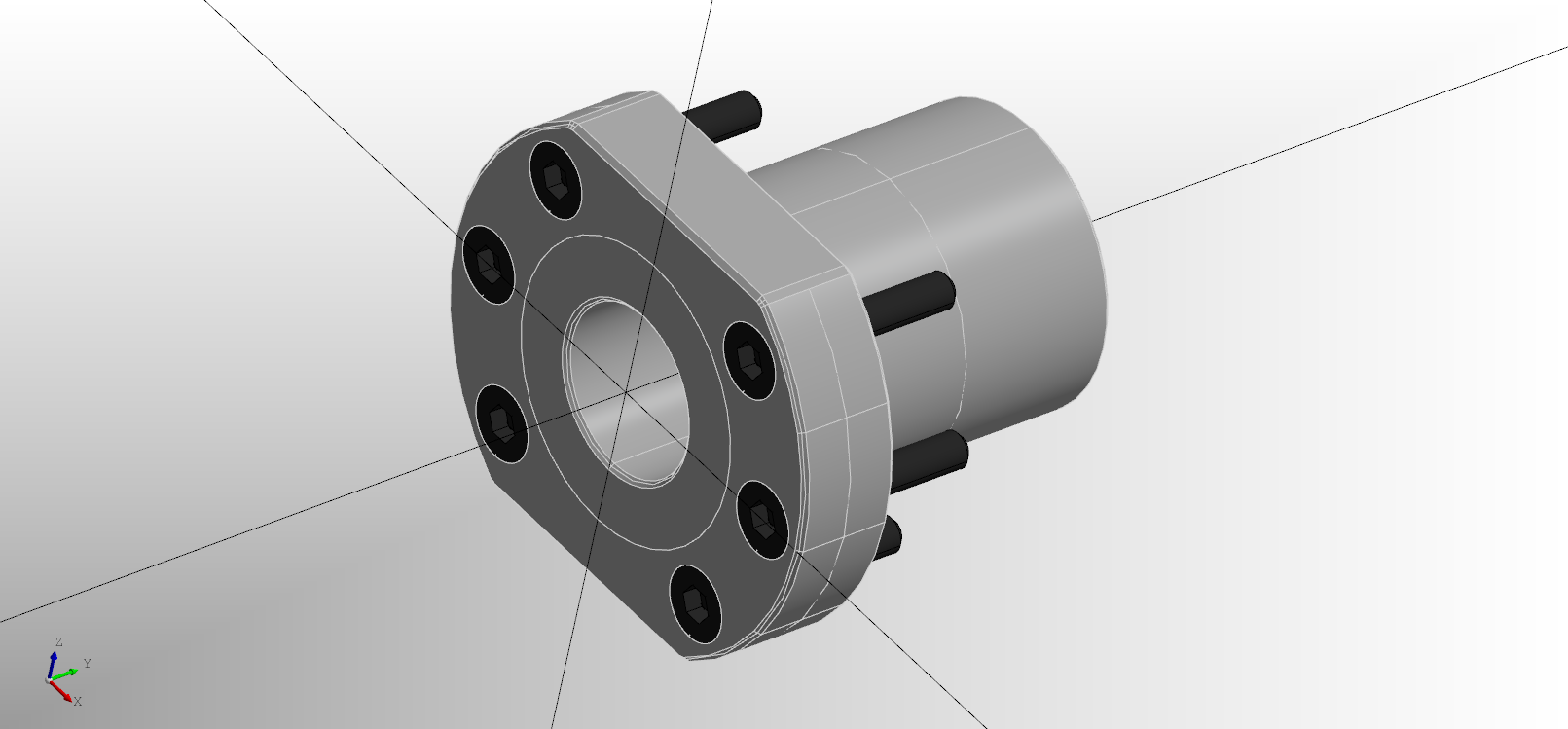``````enamldef BallScrewNut(Part): part:
attr d = 16
attr length = 42
material = 'steel'
color = '#555'
attr show_bolts: bool = True
func get_bolt_holes():
r = 38/2
angles = [
]
return [(r*cos(a), 0, r*sin(a)) for a in angles]
Chamfer:
material = part.material
color = part.color
distance = 0.5
Cut:
Fuse:
Cylinder: c2:
direction = (0, 1, 0)
height = length
# Bracket
Common:
Cylinder: c1:
direction = (0, 1, 0)
height = 10
Box:
position = (-dx/2, 0, -dz/2)
dy = 10
dz = 40
Cylinder:
direction = (0, 1, 0)
height = c2.height

Looper:
iterable = get_bolt_holes()
Cylinder:
position = loop.item
direction = (0, 1, 0)